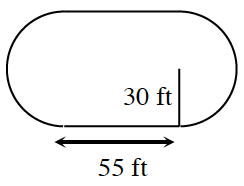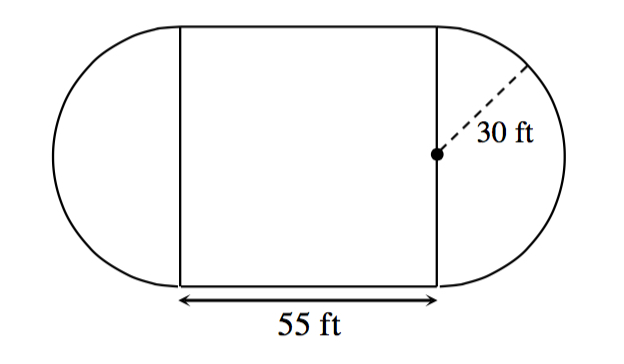### Home > INT2 > Chapter 8 > Lesson 8.4.3 > Problem8-105

8-105.The city of Denver wants you to help with their plan to build a dog park. The design of the park is a rectangle with two semicircular ends. (Note: A semicircle is half of a circle.)

1. The entire park needs to be covered with grass. If grass is sold by the square foot, how much grass should you order?

Divide the park into three regions, as shown at right. There is one rectangle and there are two half-circles.area of dog park = area of rectangle + area of circle

2. The park also needs a fence for its perimeter. A sturdy chain-link fence is sold by the foot and costs $\8$ per foot. How much will a chain-link fence for the entire park cost?

perimeter of the dog park = perimeter of semicircle + $55$ + perimeter of semicircle + $55$
= perimeter of circle + $110$

After determining the perimeter, multiply your answer by $\8$ to determine the cost.

3. The local design board has rejected the plan because it is too small. “Big dogs need lots of room to run,” the president of the board said. Therefore, you need to increase the size of the park by a linear scale factor of $2$. What is the area of the new design? What is the perimeter?

Multiply the original perimeter by $2$ and the original area by $4$. Why?